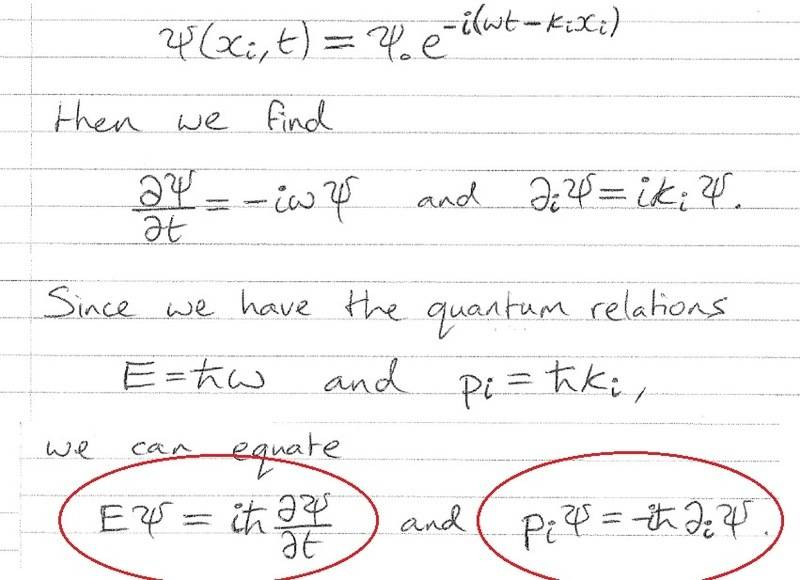# Quick derivation question Quantum Mechanics

## Homework Statement

Having one of those days where nothing makes sense, here is the derivation:how does he get the two circled terms from what he's derived above?

N/A

## The Attempt at a Solution

I tried substitution but get nowhere I just cant see how the early expressions help in getting the circled expressions.

once again, any pointers in the right direction would be appreciated

Er...isn't it just a matter of multiplying both sides by $i \hbar$?
Er...isn't it just a matter of multiplying both sides by $i \hbar$?